# Week 13: April 22: Visualization Critique / Breadboards + Speculation

### Itinerary

• Visualization Critique
• Lighting up LEDs
• Button Exercise
• Physical Interactions with the screen – Processing to Arduino
• Screen to Physical Interaction screen – Arduino to Processing
• Studio Time

### Visualization Critique

Order of Critique: Nick -Katie – Weronika – Remy – Matt – Grace – Krystal – Gabriella – Josie – Shreya – Eric – Kyndall – Ariel – Maggie

We’ll be using breadboards to help prototype our objects/designs. These allow us to connect circuits together without having to solder them permanently.The strips on the outside go continuously down, this would be a good place to plug in 5V on the +, or positive side. On the – side, you could plug in GND, or ground.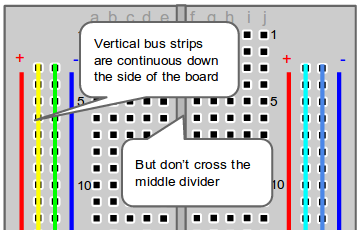The holes in abcde all go horizontally, but not across the divider.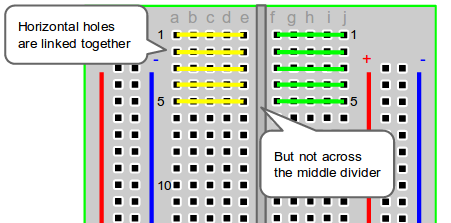### How circuits work

When working with electricity, we have to think about the creation of circuits. This means sometimes things are on, sometimes they are off, and sometimes they are given a variable amount of voltage/energy.

When we connect objects to a power source, there is a flow of energy which is measured in volts.From Wikipedia:

In the water-flow analogy, sometimes used to explain electric circuits by comparing them with water-filled pipes, voltage (difference in electric potential) is likened to difference in water pressure. Current is proportional to the diameter of the pipe or the amount of water flowing at that pressure. A resistor would be a reduced diameter somewhere in the piping.

The relationship between voltage and current is defined (in ohmic devices like resistors) by Ohm’s law. Ohm’s Law is analogous to the Hagen–Poiseuille equation, as both are linear models relating flux and potential in their respective systems.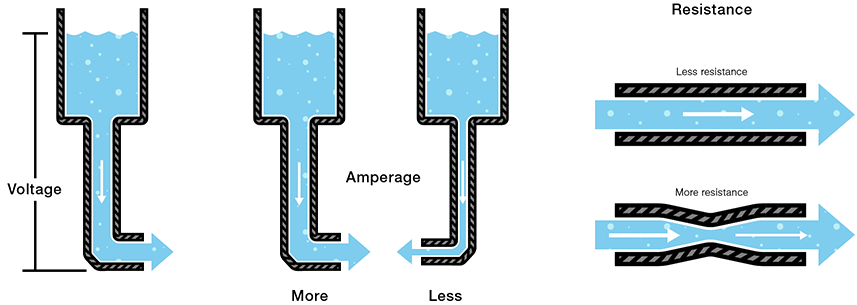In the example of the LED’s, we are creating a circuit between the LED and the Arduino. We connect the negative (or cathode) end of the LED to GND (ground/negative) of the Arduino. We connect the positive end of the LED (or anode) to one of the numbered ports of the Arduino where we then program a specific voltage to run through the LED at a distinct pattern/timing/amount.  But we are getting ahead of ourselves, more details below.

### Arduino

An Arduino is a microcontroller platform that allows us to use a simple coding interface to control hardware, sensors, and interactions with the screen.An Arduino has pins/ports by which we can control and connect sensors. The ones labeled digital can be either an input or an output – pins 2 – 13.  The Analog In pins – A0 – A5 – allow us to take readings from analog sensors on a scale of 0-1024.

The GND pin you can think of as negative, or -. The 5V pin you can think of as +, or positive. Some sensors will tell you to use either 3.3V or 5V, make sure you look specifically at which each requires.

### Setting up your Arduino Code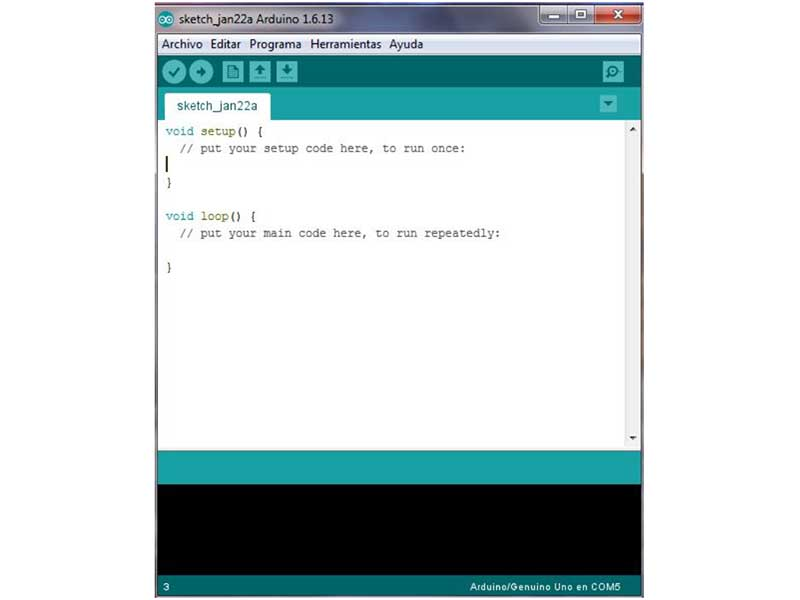A basic sketch will look like this:

```void setup() {
// put your setup code here, to run once:

}

void loop() {
// put your main code here, to run repeatedly:

}```

Familiar right? Instead of function draw, we use void loop(){ }

If we want to activate a pin to lightup or receive info, we need to initalize it in setup using pinMode
which takes two variables, the pin and the mode, which needs to either be an INPUT or OUTPUT. Its easier to create a variable at the top of the sketch to store the value of the Pin and then put that into pinMode()

```int ledPin = 13;

void setup() {
pinMode(ledPin, OUTPUT);
}```

Next, we need to initialize the serial Monitor. The serial monitor allows us to read the data coming in from Arduino and see if our sketch is working. This is also how Processing will communicate with Arduino. The serial Monitor works by passing information using an I2C protocol along the USB connection. We initialize the serial Monitor by calling Serial.begin(9600); in setup. 9600 is the baud rate, and this is standard.

```int ledPin = 13;

void setup() {
pinMode(ledPin, OUTPUT);
Serial.begin(9600);
}```

### digitalWrite() and analogWrite()To activate the blinking of an LED, we would use digitalWrite, which takes two parameters, the pin and the state. For example:

```void loop(){
digitalWrite(ledPin, HIGH);
}```

This turns the ledPin on.

```void loop(){
digitalWrite(ledPin, LOW);
}```

This turns the ledPin off.

To create timing changes, we can use delay. This takes a millisecond value (1000 milliseconds = 1 second).

`delay(500);`

This is a half second delay. Below is creating the whole blink.

```void loop(){
digitalWrite(ledPin, HIGH);
delay(500);
digitalWrite(ledPin, HIGH);
delay(500);
}```

In order to debug – we use println() to send information to the serial monitor. println() does a hard return after each line, while print() does everything on the same line. So if we wanted to see the information in the serial monitor if the LED was on or off, we would do this:

```void loop(){
digitalWrite(ledPin, HIGH);
println("led is ON");
delay(500);
digitalWrite(ledPin, HIGH);
println("led is OFF")
delay(500);
}```

We can also send specific values to the LED or other object. Instead of digitalWrite, we would use analogWrite().  analogWrite takes two parameters, the pin and the value you are sending – always between 0-255.

`analogWrite(ledPin, 100);  //medium brightness`

In the example of creating a circuit with an LED and an Arduino, we use digitalWrite we can tell the LED to change state from either off or on. When we use analogWrite, we can tell the LED to change voltage in specific amounts, to get a gradient of increasing or decreasing values.  We can use analogWrite to change the specific brightness of an LED, the speed of a motor, or the loudness of a speaker.You can only use analogWrite when you see the sign next to the number of the port you want to use. This indicates it is available for pulse width modulation / PWM – these are pins 11, 10, 9, 6, 5, and 3Sometimes we are attaching inputs that we need to receive information from to make a change, for example a Potentiometer. These take readings from 0-1024. To intialize, we have to first setup pinMode to INPUT and attach the potentiometer to an analog in.

```int potPin= A0;

void setup() {
pinMode(potPin, INPUT);
Serial.begin(9600);
}```

Next – we need to take the reading in loop, and store this in a variable

```void loop() {
println(sensor);
delay(10);
}```

We store the analogRead as an integer, and then use println to send it to the serial monitor. We use a bit of a delay in order to give the sensor a little bit of time between readings.  If we wanted to rescale this value to change the brightness of an LED, we would use map, just like we did for p5.js.

```int potPin= A0;
int ledPin = 13;

void setup() {
pinMode(potPin, INPUT);
pinMode(ledPin, OUTPUT);
Serial.begin(9600);
}

void loop() {
int mapSensor = map(sensor, 0,1024, 0,255) //rescale value
analogWrite(ledPin, mapSensor);  //use this to change the brightness value of an LED
println(sensor);
delay(10);
}```

This is the beginning of the basics of connecting sensors together.

### Button Exercise

See if you and your group can follow along and do this exercise – 30 minutes.

Challenges:

• Can you get this to work with two LEDs
• Could you do the same thing with only one button (see state change below for hint)?
• Can you use one button to increase the delay of a blink, and one to reset it?

### State Change

Sometimes we need to change the state of something, but we want to simplify how this is written. We can use an if statement to help change the condition each time the program runs. Since we are using draw here, which loops constantly, every time the program runs the condition will be the opposite.

```boolean test = false; //initialize variable up at the top

void setup() {
size(200, 200);
frameRate(2); // to slow down the animation
}

void draw() {
if (test==true) {   // check to see if test is true or false
background(255);  // white background
test =false;      // change value of change
} else {
background(0);    //black background
test = true;      // change value of change
}
}```

We could use these same conditions to simplify the blinking of an LED. Before, we turned off an on an LED by making a delay setting after each call of digitalWrite.

```digitalWrite(ledPin, HIGH);
delay(1000);
digitalWrite(ledPin, LOW);
delay(1000);```

Instead, we can use a state machine to change if the LED is on or off and only run delay once

```boolean test = false; //initialize variable up at the top
int ledPin = 13;   //set led Pin
int dTime = 1000;  // create variable for delay

void setup() {
pinMode(ledPin, INPUT);
Serial.begin(9600);
}

void loop() {
if (test==true) { // check to see if test is true or false
digitalWrite(ledPin, HIGH);
test =false; // change value of change
} else {
digitalWrite(ledPin,LOW);
test = true; // change value of change
}
delay(dTime);
}```

### Sensor Guides

Using a Photocell – Light SensorUsing a piezo buzzer – playing a scaleButtonsPotentiometer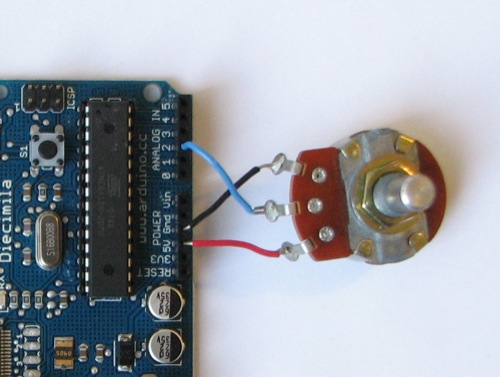Touch Sensor### Processing differences from p5.js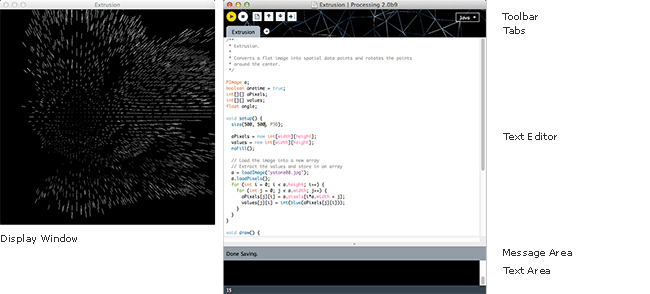So far this semester we’ve been using p5.js for creative coding. p5.js is written in JavaScript and it is the sibling/relative of Processing, which came first and is coded in Java. They are functionally and programatically similar, but there are some distinct differences in terms of syntax. For example, javascript is more forgiving if you forget a semicolon at the end of a statement. Processing and Arduino, will throw you an error. Some other differences:

To declare setup or draw, we use void instead of function.

```void setup(){
}
void draw(){
}```

You have to declare a data type. In p5.js, we used

```let x = 0;
let y = 100.101
let col = color(255,0,0);
let name = "Bobert";```

In Processing and Arduino, we have to be specific:

```int x = 0;
float y = 100.101
color col = color(255,0,0);
String name = "Bobert";```

In Processing, we declare a screen size by using:

`size(500,500);`

In Processing and Arduino we declare a for loop by:

```for(int i =0; i <10; i++){

}```

Notice that we use int i, rather than let i. That is because we are declaring the data type here. There are some other select differences, but these are the main ones.

### Arduino to Processing / Processing to Arduino

Important note: You cannot have the Serial Monitor open at the same time you have a Processing Sketch running (play button). You also cannot upload new sketches to the Arduino if you have the Processing sketch running (play button). This happens because they are both communicating on the USB cable and serial monitor – and they cannot try to do both of these at the same time.

In order to communicate from Arduino to the screen or the screen to Arduino we have to learn how to use the serial monitor a little more closely.

Challenges

• Can you get the square to move based on the value of the potentiometer
• Can you replace the square with an image from the internet ?
• Use a PNG so it has a transparent background, for example, transparent turtle:### Studio

Get into your groups and start brainstorming ideas. Sketch out on paper what you want your object for Project 3 to do.

### Homework

• 6 images to Are.na about circuits
• Continue working on Project 3, come in next week with sketches and the foundations of a website for your project
• Update /fix issues on your portfolio. The final review/critique for this course on May 6th will re-evaluate your work this semester, so this is your opportunity to fix anything that needs improvement (broken links, better composition, etc).